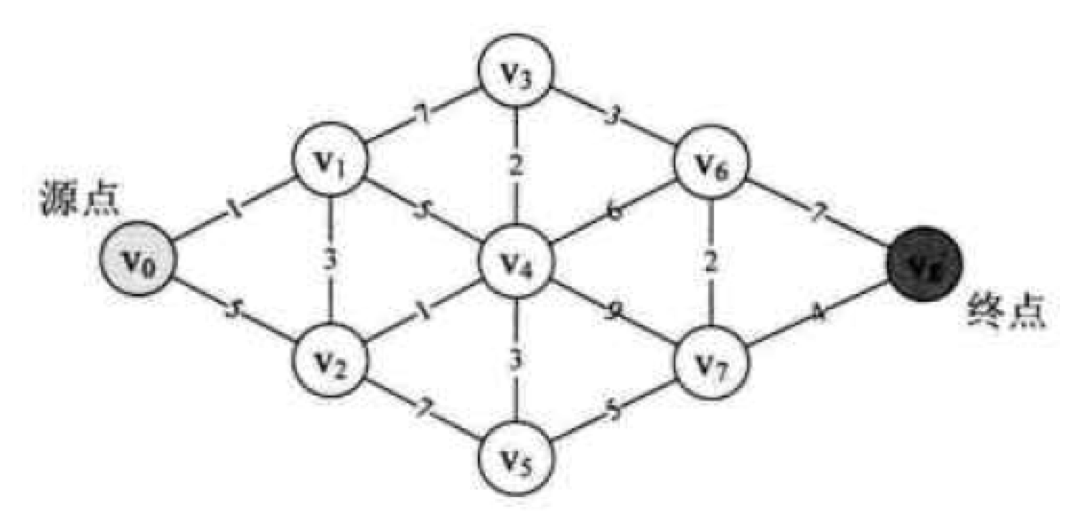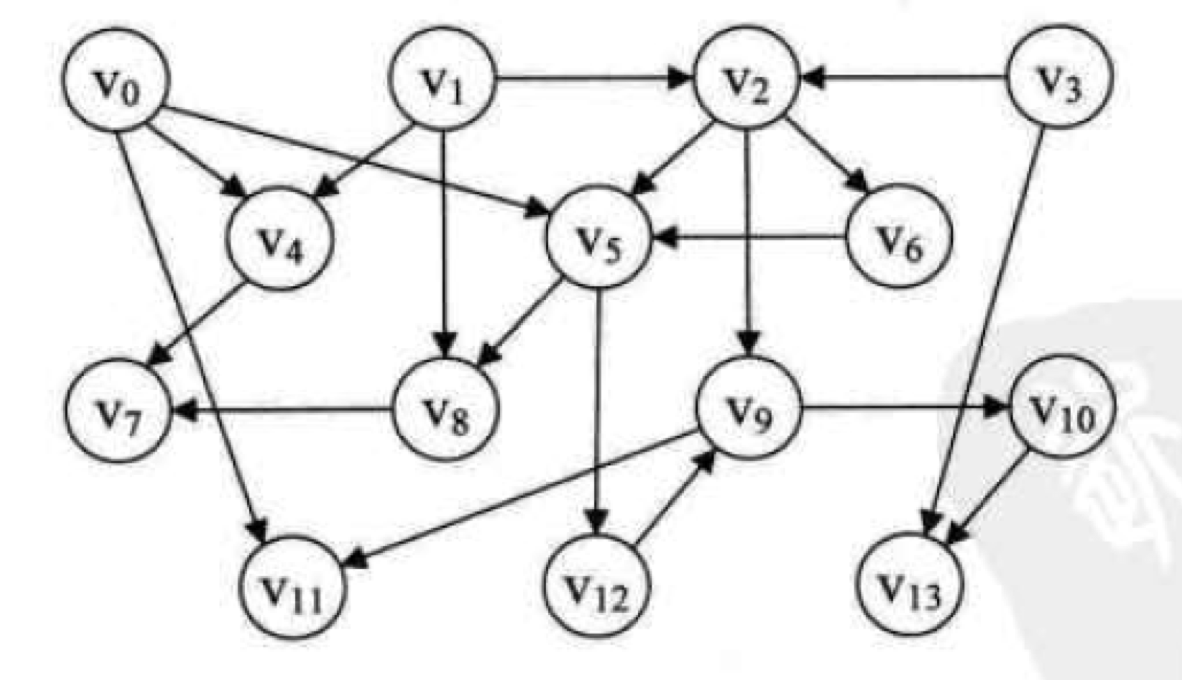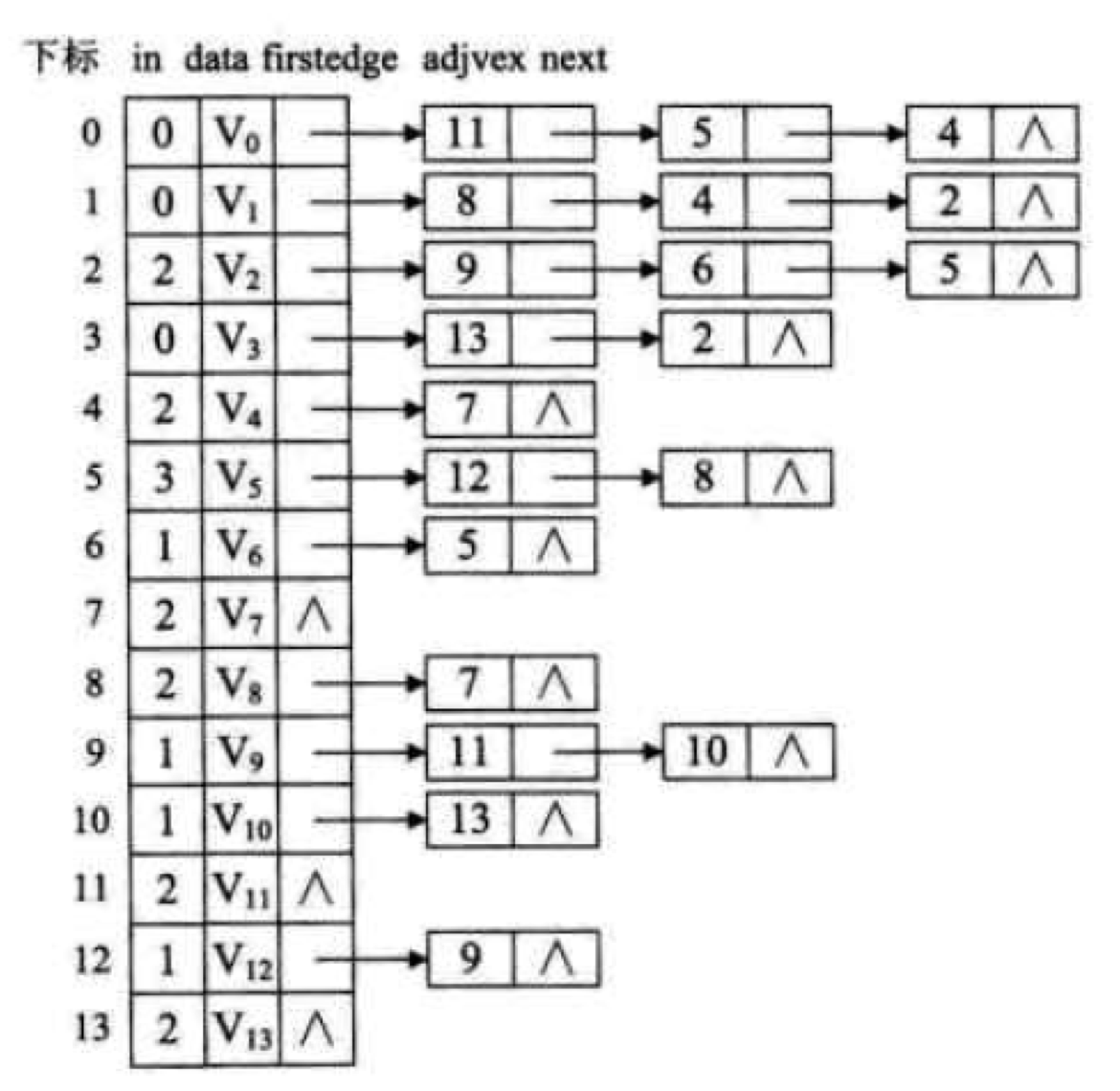• 拓扑排序 迪杰斯特拉
2019-08-13 22:04:30

遇到floyd优化了也时间超限的题
只会floyd很难受
又去学了迪杰斯特拉算法
其实和最下生成树prim基本差不多
就是遍历做标记然后循环，把最短的记录下来，遇到更短的就换掉

还有拓扑用数组容量不够了100000*100000
改用用链表解决了
把二维数组换成 一个结构体数组然后再用指针不断地指子项这样的

更多相关内容
• 例如：上图中v0-v8有9个点，可以看做不同的地点，现在要规划出v0到其它某个点地点的最短路线规划构建最短路径中比较常见的一种算法即为dijstra(迪杰斯特拉)算法二、dijstra(迪杰斯特拉)算法算法思路：dijstra算法...

一、图的最短路径从某顶点出发，沿图的边到达另一顶点所经过的路径中，各边上权值之和最小的一条路径叫做最短路径图的最短路径有许多重要的应用。

例如：上图中v0-v8有9个点，可以看做不同的地点，现在要规划出v0到其它某个点地点的最短路线规划

构建最短路径中比较常见的一种算法即为dijstra(迪杰斯特拉)算法

二、dijstra(迪杰斯特拉)算法

算法思路：

dijstra算法思路有点类似前一篇文章中的prim算法，先构建图的邻接矩阵，然后定义一个临时的一维数组，一维数组用来存储v0到每个顶点的最短路径

将一维数组初始化成v0到每个顶点的值。其次定义另外一个数组用来存储已经访问过的顶点

在临时一维数组中找到未被访问且最短的一条路径

将找到最短路径的顶点与该顶点可访问边进行遍历，若最短路径与边之和小于一维数组中存储的最短路径，则将这个和覆盖成这个顶点的最短路径

循环3，4直至遍历完所有顶点为止

构建邻接矩阵

1

2

3

4

5

6

7

8

9

10// 初始化图

int[] graph0 = new int[]{0, 1, 5, MAX_WEIGHT, MAX_WEIGHT, MAX_WEIGHT, MAX_WEIGHT, MAX_WEIGHT, MAX_WEIGHT};

int[] graph1 = new int[]{1, 0, 3, 7, 5, MAX_WEIGHT, MAX_WEIGHT, MAX_WEIGHT, MAX_WEIGHT};

int[] graph2 = new int[]{5, 3, 0, MAX_WEIGHT, 1, 7, MAX_WEIGHT, MAX_WEIGHT, MAX_WEIGHT};

int[] graph3 = new int[]{MAX_WEIGHT, 7, MAX_WEIGHT, 0, 2, MAX_WEIGHT, 3, MAX_WEIGHT, MAX_WEIGHT};

int[] graph4 = new int[]{MAX_WEIGHT, 5, 1, 2, 0, 3, 6, 9, MAX_WEIGHT};

int[] graph5 = new int[]{MAX_WEIGHT, MAX_WEIGHT, 7, MAX_WEIGHT, 3, 0, MAX_WEIGHT, 5, MAX_WEIGHT};

int[] graph6 = new int[]{MAX_WEIGHT, MAX_WEIGHT, MAX_WEIGHT, 3, 6, MAX_WEIGHT, 0, 2, 7};

int[] graph7 = new int[]{MAX_WEIGHT, MAX_WEIGHT, MAX_WEIGHT, MAX_WEIGHT, 9, 5, 2, 0, 4};

int[] graph8 = new int[]{MAX_WEIGHT, MAX_WEIGHT, MAX_WEIGHT, MAX_WEIGHT, MAX_WEIGHT, MAX_WEIGHT, 5, 4, 0};

算法代码

1

2

3

4

5

6

7

8

9

10

11

12

13

14

15

16

17

18

19

20

21

22

23

24

25

26

27

28

29

30

31

32

33

34

35

36

37

38

39

40

41

42/**

* 图的最短路径(图中两个顶点的最短距离)，迪杰斯特拉算法

* 算法思想：类似prim算法，定义一个一维数组，用来存储V0到某点的最短路径

*/

public void shortestPathDijstra() {

// 定义一维数组用来存储V0到每个点的最短路径，找到比原来更短的则直接覆盖

int[] paths = new int[vertexSize];

// 先将数组初始化

System.arraycopy(matrix, 0, paths, 0, vertexSize);

isVisited = true;

for (int i = 1; i < vertexSize; i++) {

// 在已经存在的路径中找到一条未被访问且最短的路径

int min = MAX_WEIGHT;

int minIndex = -1;

for (int j = 1; j < vertexSize; j++) {

if (!isVisited[j] && paths[j] < min) {

min = paths[j];

minIndex = j;

}

}

if (minIndex == -1) {

continue;

}

isVisited[minIndex] = true;

// 找到的最短路径节点的可使用边中，判断是否比已经存在的最短路径短，是则进行覆盖

for (int k = 1; k < vertexSize; k++) {

if (!isVisited[k] && (min + matrix[minIndex][k] < paths[k])) {

paths[k] = min + matrix[minIndex][k];

}

}

}

for (int i = 1; i < vertexSize; i++) {

System.out.println("V0到V" + i + "的最短路径为：" + paths[i]);

}

}

即可得到v0到每个点的最短路径的值三、拓扑排序对一个有向无环图(Directed Acyclic Graph简称DAG)G进行拓扑排序，是将G中所有顶点排成一个线性序列，使得图中任意一对顶点u和v，若边(u,v)∈E(G)，则u在线性序列中出现在v之前。通常，这样的线性序列称为满足拓扑次序(Topological Order)的序列，简称拓扑序列。

简单的说，由某个集合上的一个偏序得到该集合上的一个全序，这个操作称之为拓扑排序。

对以下有向图进行拓扑排序，顶点可以看待成每项不同的任务，有的任务可以直接执行，例如v0、v1、v3，有的任务得等到上级任务全部执行完成才可执行，例如v4必须等到v0和v1都完成之后才可执行排序思路：

观察可以发现顶点入度为0则代表可以直接执行

完成一个任务之后可消除出度的边，即他的next节点则少了一个入度

带next节点入度也为0之后就可执行

循环1，2，3遍历完所有的顶点即可排序完成

排序代码：

构建每个顶点的邻接表算法代码

1

2

3

4

5

6

7

8

9

10

11

12

13

14

15

16

17

18

19

20

21

22

23

24

25

26

27

28

29

30

31

32/**

* 拓扑排序

* 思路：定义一个栈，存放入度为0的节点，入度为0则代表可以执行，执行之后则出度的那个节点就减少一个入度，循环直至栈为空

*/

private void topologicaSort() {

Stack stack = new Stack<>();

// 先将入度为0 的节点压入栈中

for (int i = 0; i < adjList.length; i++) {

stack.push(i);

}

}

int count = 0;

// 循环出栈输出，直至栈为空

while (!stack.isEmpty()) {

int index = stack.pop();

count++;

for (EdgeNode edgeNode = adjList[index].firstEdge; edgeNode != null; edgeNode = edgeNode.next) {

// 若输出之后的顶点入度也没0，则压入栈中

}

}

}

if (count != numVertexes) {

System.out.println("拓扑排序失败！！！！");

}

}

展开全文• 再输入那P条单向边，现在就变成了一个有向无环图，我们一边拓扑排序一边迪杰斯特拉，连有向边的时候用一个数组计算每个强连通分量的入度，将入度为0的点塞进一个队列中，然后对队列内的点挨个跑迪杰斯特拉迪杰...

# 道路与航线

## 题目描述：

n个点，R条双向边，P条单向边，双向边的权值都是正的，单向边的权值有正有负，给你一个起点，问起点到每个点的最短路是多少

## 思路1：

第一眼就看出来是SPFA板子题，但是敲上去发现SPFA被卡死了，但是用SLF优化一下SPFA就过了

思想是：将队列换成双端队列，塞点的时候先和队首比较一下，如果dis小于队首的dis，就把他塞到队首，否则塞到队尾

#include <bits/stdc++.h>
using namespace std;

#define endl '\n'
#define inf 0x3f3f3f3f
#define mod 1000000007
#define m_p(a,b) make_pair(a, b)
#define mem(a,b) memset((a),(b),sizeof(a))
#define io ios::sync_with_stdio(false); cin.tie(0); cout.tie(0)

typedef long long ll;
typedef pair <int,int> pii;

#define MAX 300000 + 50
int n, m1, m2;
int s;
int a, b, c;

int tot;
struct ran{
int to, nex, val;
}tr[MAX];
inline void add(int u, int v, int c){
tr[++tot].to = v;
tr[tot].val = c;
}
int dis[MAX];
bool vis[MAX];
void SPFA(){
deque<int>q;q.push_back(s);vis[s] = 1;
mem(dis, inf);dis[s] = 0;
while (!q.empty()) {
int u = q.front();q.pop_front();vis[u] = 0;
for(int i = head[u]; i; i = tr[i].nex){
int v = tr[i].to;
if(dis[v] > dis[u] + tr[i].val){
dis[v] = dis[u] + tr[i].val;
if(!vis[v]){
vis[v] = 1;
if(q.empty() || dis[v] > dis[q.front()])q.push_back(v);
else q.push_front(v);
}
}
}
}
for(int i = 1; i <= n; ++i){
if(dis[i] == inf)cout << "NO PATH\n";
else cout << dis[i] << endl;
}
}

void work(){
cin >> n >> m1 >> m2 >> s;
for(int i = 1; i <= m1; ++i){
cin >> a >> b >> c;
}
for(int i = 1; i <= m2; ++i){
cin >> a >> b >> c;
}
SPFA();
}

int main(){
io;
work();
return 0;
}



## 思路2:

如果SPFA怎么优化都过不去的话怎么办？

题面最有意思的一个点是双向边的都是正权值，单向边的都是负权值，而我们不用迪杰斯特拉的原因是它不能跑负权值，那我们可以先将双向边的那些点进行缩点操作，因为是双向边，所以肯定会将原图变成一个个强连通分量，把每个强连通分量看成一个新点，这是图论中连通性问题常见的用法。再输入那P条单向边，现在就变成了一个有向无环图，我们一边拓扑排序一边迪杰斯特拉，连有向边的时候用一个数组计算每个强连通分量的入度，将入度为0的点塞进一个队列中，然后对队列内的点挨个跑迪杰斯特拉，迪杰斯特拉塞点之前先判断一下vu是不是在一个连通块内，如果是才可以塞进去。如果vu不在一个连通块内，记得更新v所在的连通块的入度，入度为0时要及时入队

#include <bits/stdc++.h>
using namespace std;

#define endl '\n'
#define inf 0x3f3f3f3f
#define mod 1000000007
#define m_p(a,b) make_pair(a, b)
#define mem(a,b) memset((a),(b),sizeof(a))
#define io ios::sync_with_stdio(false); cin.tie(0); cout.tie(0)

typedef long long ll;
typedef pair <int,int> pii;

#define MAX 300000 + 50
int n, m1, m2, s;
int a, b, c;

int num;
struct ran{
int to, nex, val;
}tr[MAX];
inline void add(int u, int v, int c){
tr[++num].to = v;
tr[num].val = c;
}

vector<int>v[MAX];
int tot;
int id[MAX];
void dfs(int u){
id[u] = tot;
v[tot].push_back(u);
for(int i = head[u]; i; i = tr[i].nex){
int v = tr[i].to;
if(!id[v])dfs(v);
}
return;
}
queue<int>qq;
int du[MAX];
int dis[MAX];
bool vis[MAX];
void dijkstra(int p){
priority_queue<pii, vector<pii>, greater<pii>>q;
for(auto x : v[p])q.push(m_p(dis[x], x));
while (!q.empty()) {
auto [d, u] = q.top();q.pop();
if(vis[u])continue;
vis[u] = 1;
for(int i = head[u]; i; i = tr[i].nex){
int v = tr[i].to;
if(dis[v] > dis[u] + tr[i].val){
dis[v] = dis[u] + tr[i].val;
if(id[v] == p)q.push(m_p(dis[v], v));
}
if(id[v] != p){
--du[id[v]];
if(!du[id[v]])qq.push(id[v]);
}
}
}
}

void work(){
cin >> n >> m1 >> m2 >> s;
for(int i = 1; i <= m1; ++i){
cin >> a >> b >> c;
}
for(int i = 1; i <= n; ++i){
if(!id[i]){
++tot;
dfs(i);
}
}
for(int i = 1; i <= m2; ++i){
cin >> a >> b >> c;
++du[id[b]];
}
for(int i = 1; i <= tot; ++i){
if(!du[i])qq.push(i);
}
mem(dis, inf);
dis[s] = 0;
while (!qq.empty()) {
dijkstra(qq.front());qq.pop();
}
for(int i = 1; i <= n; ++i){
if(dis[i] > inf / 2)cout << "NO PATH\n";
else cout << dis[i] << endl;
}
}

int main(){
io;
work();
return 0;
}

展开全文算法
• 思路：根据题意我们可以吧双向的道路变成团，用航道连接不同的团形成一个DAG图，然后我们按拓扑排序去跑每个团里的迪杰斯特拉。 #pragma GCC optimize(2) #include using namespace std; typedef long long ll; #...## 思路：根据题意我们可以吧双向的道路变成团，用航道连接不同的团形成一个DAG图，然后我们按拓扑排序去跑每个团里的迪杰斯特拉。

#pragma GCC optimize(2)
#include<bits/stdc++.h>

using namespace std;
typedef long long ll;
#define SIS std::ios::sync_with_stdio(false)
#define space putchar(' ')
#define enter putchar('\n')
#define lson root<<1
#define rson root<<1|1
typedef pair<int,int> PII;
typedef pair<int,PII> PIII;
const int mod=1e9+7;
const int N=2e5+5;
const int inf=0x7f7f7f7f;

int gcd(int a,int b)
{
return b==0?a:gcd(b,a%b);
}

ll lcm(ll a,ll b)
{
return a*(b/gcd(a,b));
}

template <class T>
{
char c;
bool op = 0;
while(c = getchar(), c < '0' || c > '9')
if(c == '-')
op = 1;
x = c - '0';
while(c = getchar(), c >= '0' && c <= '9')
x = x * 10 + c - '0';
if(op)
x = -x;
}
template <class T>
void write(T x)
{
if(x < 0)
x = -x, putchar('-');
if(x >= 10)
write(x / 10);
putchar('0' + x % 10);
}
ll qsm(int a,int b,int p)
{
ll res=1%p;
while(b)
{
if(b&1)
res=res*a%p;
a=1ll*a*a%p;
b>>=1;
}
return res;
}
struct node
{
int to,nex,w;
}edge[N];
int tot,bcnt;
int n,tr,tp,s;
vector<int> block[N];
int dis[N],vis[N];
queue<int> q;
{
edge[tot].to=v;
edge[tot].w=w;
}

void dfs(int u,int bid)
{
id[u]=bid;
block[bid].push_back(u);
{
int v=edge[i].to;
if(!id[v])dfs(v,bid);
}

}
void dij(int x)
{
priority_queue<PII,vector<PII>,greater<PII> >heap;
for(auto v:block[x])heap.push({dis[v],v});
while(heap.size())
{
auto now=heap.top();heap.pop();
int distance=now.first,ver=now.second;
if(vis[ver])continue;
vis[ver]=1;
{
int v=edge[i].to,w=edge[i].w;
if(dis[v]>dis[ver]+w)
{
dis[v]=dis[ver]+w;
if(id[v]==id[ver])heap.push({dis[v],v});
}
if(id[v]!=id[ver]&&--din[id[v]]==0)q.push(id[v]);
}

}
}
void topusort()
{
memset(dis,inf,sizeof dis);
dis[s]=0;
for(int i=1;i<=bcnt;i++)
{
if(!din[i])q.push(i);
}
while(q.size())
{
int t=q.front();q.pop();
dij(t);
}

}

int main()
{
scanf("%d%d%d%d",&n,&tr,&tp,&s);
while(tr--)
{
int a,b,c;
scanf("%d%d%d",&a,&b,&c);
}

for(int i=1;i<=n;i++)
{
if(!id[i])dfs(i,++bcnt);
}
while(tp--)
{
int a,b,c;
scanf("%d%d%d",&a,&b,&c);
din[id[b]]++;
}
topusort();

for(int i=1;i<=n;i++)
{
if(dis[i]>inf/2)puts("NO PATH");
else printf("%d\n",dis[i]);
}

return 0;

}



## 思路：SPFA的优化

#include<bits/stdc++.h>
using namespace std;
typedef long long ll;
const int N=200005;
const int mod=1e9+7;
const int M=(1<<10)+5;
const int inf=0x3f3f3f3f;
int n,T,R,S;
struct node
{
int to,nex,w;
}edge[N];
{
edge[++tot].to=v;
edge[tot].w=w;

}
void spfa()
{
memset(dis,inf,sizeof dis);
deque<int> q;
dis[S]=0;
q.push_back(S);
vis[S]=1;
while(!q.empty()){
int x=q.front();
q.pop_front();
vis[x]=0;
{
int v=edge[i].to;
if(dis[v]>dis[x]+edge[i].w){
dis[v]=dis[x]+edge[i].w;
if(!vis[v]){
vis[v]=1;
if(q.empty()) q.push_back(v);
else{
if(dis[v]<dis[q.front()])q.push_front(v);
else q.push_back(v);
}
}
}
}
}

}
int main()
{
scanf("%d%d%d%d",&n,&T,&R,&S);
for(int i=0;i<T;i++)
{
int u,v,w;
scanf("%d%d%d",&u,&v,&w);
}
for(int i=0;i<R;i++)
{
int u,v,w;
scanf("%d%d%d",&u,&v,&w);
}
spfa();
for(int i=1;i<=n;i++)
{
if(dis[i]!=inf){
printf("%d\n",dis[i]);
}
else{
printf("NO PATH\n");
}
}
return 0;

}


展开全文• 它和上一篇介绍到的拓扑排序一样属于图论算法，是大学计算机专业必学的算法之一，也是笔试经常出的算法。那么下面让我们一起来学习迪杰斯特拉算法的实现。 算法思想 迪杰斯特拉算法需要维护以下两张列表： visited...算法 java
• 拓扑排序核心算法  在一个有向图中找到一个拓扑排序的过程如下：  1）从一个有向图中选择一个没有前驱(入度为0)的顶点输出；  2）删除1)中的顶点，并删除从该顶点出发的全部边；  3）重复上述两步，直到剩余的图...算法
• 拓扑排序： 应用于DAG图。先遍历一遍（DFS、BFS），每个节点标记入度（in-degree）。入度 为0的节点，放入一个队列。 初始时，该队列中的元素都是第一批的点（不依赖于任何其他元素）； 之后，弹出该队列中所有...
• 迪杰斯特拉算法 该算法设置一个集合S记录已求得的最短路径的顶点，可用一个数组s[]来实现，初始化为0，当s[vi]=1时表示将顶点vi放入S中，初始时把源点v0放入S中。此外，在构造过程中还设置了两个辅助数组： dist...
• 文章内容概览迪杰斯特拉算法Dijkstra(迪杰斯特拉)算法是著名的图论算法Dijkstra算法解决有权图从一个节点到其它节点的最短路径问题特点：“以起点为中心，向外层层扩展”最短路径问题假设有下图这样的一个网络，该...
• （4）图的拓扑排序：图的BFS的应用题 （5）图的最小生成树：Kruskal算法–并查集的经典应用，解决连通性问题 （6）图的最小生成树：Prim算法–解锁一批边，解锁一批节点 文章目录 图的最小生成树：Dijkstra迪杰...dijkstra算法 最小生成树 连通性 数据结构与算法
• 目前，单源最短路径比较好的算法有迪杰斯特拉算法（贪心算法，效率最高，局限：图中不可有负权边），贝尔曼-福特算法（可以判断能否求出最短路径并找出负权环，但速度比迪杰斯特拉算法慢）。多源最短路径有弗洛伊德...算法 单源最短路径
• 拓扑排序 并查集 多源最短路径（弗洛伊德算法） 单源最短路径（迪杰斯特拉算法） 其中呢，最基本的是前两种，也就是平时常用的广搜和深搜，本文中将概要举例讲解。因为基础也很重要啊~~ 图的算法题当想...图算法 Java实现 LeetCode 并查集
• 拓扑排序 Topological sort 这个视频讲的很好还简单 拓扑排序！（自讲) 简而言之，就是每次删除入度为0的顶点和它的边，作为所求序列的下一个值。 例如 5. 迪杰斯特拉找最短路径 找图中任意一点到其他点最短路径。...数据结构 算法
• 拓扑排序的算法思想和迪杰斯特拉算法思想，浅显易懂，附代码
• 解题 先预处理出每个点 到 n 点 的最短路径，参考迪杰斯特拉算法 再建立 1 开始的最短路径是递减的 新图，同时记录节点的入度 采用 拓扑排序，累积前一个节点转移过来的方案数 typedef pair, int> pii; struct cmp{...
• 该算法是由荷兰计算机科学家迪杰斯特拉于1959年提出的。 /** * 实验题目: * 采用迪杰斯特拉算法求带权有向图的最短路径 * 实验目的: * 领会迪杰斯特拉算法求带权有向图中单源最短路径的过程和相关算法设计 * 实验...
• 拓扑排序 Q：不知道你是否也曾苦恼于生物里的求食物链数目的题目。如果现在任意给你一个食物网，你能用程序来求出这个食物网中食物链的数量吗？ A：你初次看到这个题目时，也许会不知从何下手。但如果你之前对拓扑...算法
• k"迪杰斯特拉算法-两点之间最短路径/一个节点到各个节点的最短路径 邻接矩阵 int Dij(int n,vector&edge,int start,int target){ vector>GMatrix(n,vector(n,INT_MAX)); vectordistance(n,INT_MAX);//start节点到...
• 2、克鲁斯卡尔算法 二、最短路径 1、迪杰斯特拉算法 （从某个源点到其余各顶点的距离） 时间复杂度为O(n^2) 2、弗洛伊德算法 （每一对顶点之间的最短路径） 时间复杂度为O(n^3) 三、拓扑排序 1、AOV-网 用顶点表示...数据结构 有向图
• 1.有向图：比较好 2.无向图是变态的有向图，需要注意度和节点之类的比如MST，最小...1.用拓扑分类算法，找到图中的圈。具体就是依次找到图中度为1的顶点（可以保存在队列里），删除之（这里的删除是暂时的，下次遍历算法
• 首语 上一篇：数据结构与算法（图的遍历与最小生成树） ...迪杰斯特拉算法(Dijkstra)是由荷兰计算机科学家狄克斯特拉于1959 年提出的，因此又叫狄克斯特拉算法。是从一个顶点到其余各顶点的最短路径算法，解...最短路径 邻接顶点
• 1) 有一网格状拓扑（25*20，数据见gridtopo.txt），现在需要组建长期运输网；拓扑中链路的最大容量（最大承受带宽）已知；链路的单位质量业务的传输成本已知； 2) 有1000种蚁穴到蚁穴（源节点到终节点）的业务需要...Floyd 动态规划 最短路径
• 网络延迟时间【模板题】 当然，我们会发现在每一次寻找 getMinDistanceAndUnselectedNode 浪费了大量的时间，基于堆优化的迪杰斯特拉算法 对此做了优化，有兴趣的读者可以去看看，这里就不再论述了 本期的内容就到...算法...